# Category Theory - Comma and Slice Categories

 "Comma categories were introduced by Lawvere  in the context of the interdefinabilty of the universal concepts of category theory. The basic idea is the elevation of arrows of one category to objects in another" - Computational Category Theory, D.E. Rydeheard R.M. Burstall.

Slice category is a specific case of comma category. To introduce the subject gradually lets start with the slice category then move on to the more complicated comma category later.

## Slice Category

Given a category C we can 'slice' it over some object XC which we fix in C.

 This construction allows us to start with one category 'C' and generate a different category 'C/X' by fixing a given element 'X' in C. The elements in C/X are pairs (A,P) where AC and P is a morphism from A to X.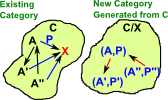'co-slice' is the dual concept, just reverse all the arrows.

#### Slice Category Examples

 Colouring of labeled set. Here we choose as our fixed object 'X' the 3 element set containing R, G and B (for red green and blue). Every object (in this case set) has an arrow (function) to this set so all the elements are assigned a colour. more hereAdding a distinguished point (the origin) to Euclidean space to give a vector space. more here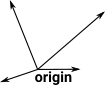## Comma Category

 In the slice category all the objects are in the same category (including the fixed object). The comma category generalises this by allowing the objects to come from other categories.### Special Cases of Comma Category

We have already seen the slice category, here is a fuller list of special cases and related constructs:

#### Slice Category

If 's' is the identity functor of C and 't' is the inclusion 1 -> C of an object cC, then (s/t) is the slice category C/c.

See ncatlab site.#### Co-slice Category

Likewise if 't' is the identity and 's' is the inclusion of c, then (t/s) is the coslice category c/C

See ncatlab site.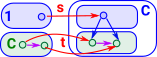#### Arrow Category

This is all derived from a single category. So it is a comma category where the arrows into it are identity functions. Unlike the slice or co-slice both the source and target can be somthing other than the terminal object.

• An object 'a' of Arr(C) is a morphism a:a0->a1 of C
• A morphism f:a->b of Arr(C) is a square.#### Graph Category

The category of graphs is an example of a comma category, that is, a graph is isomorphic to a comma category. Arrows of graphs are pairs of functions mapping nodes to nodes (N -> N) and edges to edges (E -> E) , an object of this category it a triple (E,f: E -> N×N,N) where f maps each edge to a source and target node.

See page here.

#### Universal Morphism

"The notion of a universal morphism to a particular colimit, or from a limit, can be expressed in terms of a comma category. Essentially, we create a category whose objects are cones, and where the limiting cone is a terminal object; then, each universal morphism for the limit is just the morphism to the terminal object. "- academickids.com

See page here.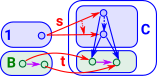"Lawvere showed that the functors F : C->D and G : D->C are adjoint if and only if the comma categories FD and $C$G $are isomorphic, and equivalent elements in the comma category can be projected onto the same element of C×D. This allows adjunctions to be described without involving sets, and was in fact the original motivation for introducing comma categories."-academickids.com$

See page here.See page here.

### DiscussionThe comma category is a specialisation of the arrow category where the codomain is the same for all objects. Alternatively there is also a cocomma category where the domain is the same for all objects.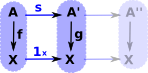The above diagram could be rewritten with the X shown in each object to make it look more like the arrow category.

The concept of a comma category is related to the idea of a fibre and sheaf, reading these pages for may help with intuition.

#### Objects

pairs(A,f)

Where:

• 'A' is any object in C
• 'f' is a mapping from A to X:
 Af X
pairs(A,f)
 Xf A

#### Morphisms

The morphism f:
 s (A,f) -> (A',f')
Such that the diagram commutesThe morphism f:
 t (A,f) -> (A',f')
Such that the diagram commutes#### Initial Objects in Co-Comma

If C has a terminal object '1' exists then:

C/1 = C

If C has an initial object '0' exists then:

0/C = C

#### CoProducts in Co-Comma

In terms of the above 'pairs' we can construct a product:
 Take arbitrary elements: A,B,D and mappings of these to X s : A->X, t : B->X, μ : D->X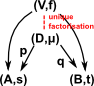We can reduce the above diagram to:## General Comma Category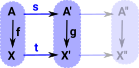The comma category can be generalised further by making the target also a functor.

### Further Genralisation

So we fix f: C->D and XD

#### Objects

pairs(cC,p:Fc->X)
 FcP X
Where
pairs(cC,p:X->Fc)
 XP Fc

#### Morphisms

 The morphism f: c->c'C Such that the diagram commutesThe morphism f: c->c'C Such that the diagram commutesWe can generalise this further by not fixing XInstead x is combined with the pair to give a triple.

#### Objects

triple(cC,xD,p)

Where:

• x is any element in D (no longer fixed)
 FcP X
triple(cC,xD,p)
 XP Fc

#### Morphisms

 The morphisms are: f: c->c'C g: x->x'D Such that the diagram commutes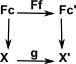Further generalisation, we add a third category 'E' like this: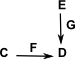#### Objects

quad(cC,eE,FX,p)
 FcP X
Where x is any element in D (no longer fixed)

#### Morphisms

 The morphisms are: f: c->c'C g: x->x'D Such that the diagram commutes### Comma Category References

Where I can, I have put links to Amazon for books that are relevant to the subject, click on the appropriate country flag to get more details of the book or to buy it from them.The Princeton Companion to Mathematics - This is a big book that attempts to give a wide overview of the whole of mathematics, inevitably there are many things missing, but it gives a good insight into the history, concepts, branches, theorems and wider perspective of mathematics. It is well written and, if you are interested in maths, this is the type of book where you can open a page at random and find something interesting to read. To some extent it can be used as a reference book, although it doesn't have tables of formula for trig functions and so on, but where it is most useful is when you want to read about various topics to find out which topics are interesting and relevant to you.